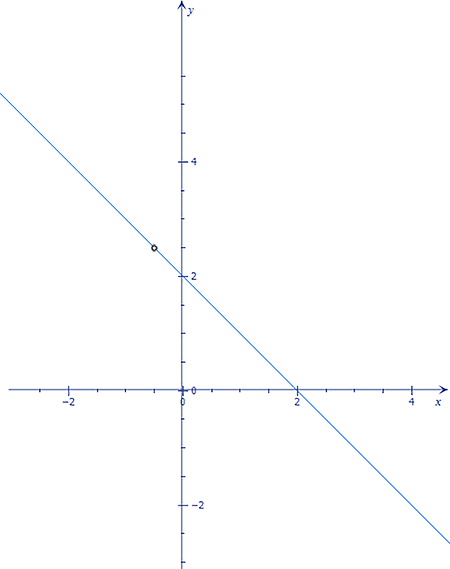# Write a complete approach statement for y = fraction {(2x + 1)(2 - x)}{2x + 1}.

## Question:

Write a complete approach statement for {eq}\displaystyle y = \dfrac{(2x + 1)(2 - x)}{2x + 1}. {/eq}

## Analyzing Rational Functions

A rational function is any function which can be written as the ratio of two polynomial functions, where the polynomial in the denominator is not equal to zero, i. e., given {eq}g(x) {/eq} and {eq}h(x) \neq 0, {/eq} the function {eq}f {/eq} defined by {eq}f(x) = \dfrac{g(x)}{h(x)} {/eq} is a rational function.

As defined above, the given function {eq}y, {/eq} is a rational function. Its domain, is the set of the {eq}x \in \mathbb{R} {/eq} where {eq}x \neq -\dfrac{1}{2} {/eq}.

We can also notice that we can rewrite {eq}y {/eq} as

$$y = \dfrac{(2x + 1)(2 - x)}{2x + 1} \implies y = \dfrac{2x + 1}{2x + 1} \cdot (2 - x),$$

and, since {eq}\dfrac{2x + 1}{2x + 1} = 1, {/eq} we have that {eq}y = (2 - x) {/eq} and its domais is given as {eq}\{x\in \mathbb{R} : x \neq -\dfrac{1}{2} \}. {/eq}

Here we have a plot of the graphic of this function